Note: This is a working paper which will be expanded/updated frequently. All suggestions for improvement are welcome. The directory deleeuwpdx.net/pubfolders/stability has a pdf version, the bib files, the complete Rmd file with the code chunks, and the R source code.

# 1 Introduction

In Individual Differences Multidimensional Scaling (IDMDS) we minimize the stress loss function, first proposed by Kruskal (1964a), Kruskal (1964b), and defined as

$\begin{equation}\label{E:stress} \sigma(X_1,\cdots,X_m)=\frac12\sum_{k=1}^m\mathop{\sum\sum}_{1\leq i<j\leq n}w_{ijk}(\delta_{ijk}-d_{ij}(X_k))^2 \end{equation}$

over the $$n\times p$$ configurations $$X_1,\cdots,X_m$$. In $$\eqref{E:stress}$$ the $$w_{ijk}$$ are fixed and known weights, the $$\delta_{ijk}$$ are fixed and known dissimilarities, and $$d_{ij}(X_k)$$ is the Euclidean distance between rows $$i$$ and $$j$$ of $$X_k$$.

The configurations are constrained to be of the form$$X_k=XT_k,$$ where $$T_k$$ is a square matrix of order $$p$$. In IDMDS we typically choose $$T_k$$ to be either a general matrix (IDIOSCAL) or a diagonal matrix (INDSCAL).

# 2 Weighted Euclidean Distances

The Euclidean distance $$d_{ij}(X_k)$$ can be expressed in various ways. Some interesting ones, for our purposes, are

\begin{align}\label{E:xdist} d_{ij}(X_k)&=\sqrt{(x_i-x_j)'C_k(x_i-x_j)}\\&=\sqrt{\mathbf{tr}\ X_k'A_{ij}^{\ }X_k^{\ }}\\&=\sqrt{x'(C_k\otimes A_{ij})x}, \end{align}

where

\begin{align*} C_k^{\ }&=T_k^{\ }T_k',\\ A_{ij}&=(e_j-e_j)(e_i-e_j)',\\ x_i&=X'e_i,\\ x&=\mathbf{vec}(X). \end{align*}

In these expressions the $$e_i$$ and $$e_j$$ are unit n-element vectors (columns of the identity matrix), with a single element equal to one and all other elements equal to zero. Thus $$A_{ij}$$ is an $$n\times n$$ matrix, with elements $$(i,i)$$ and $$(j,j)$$ equal to $$+1$$, elements $$(i,j)$$ and $$j,i)$$ equal to $$-1$$, and all other elements equal to zero. We use $$\otimes$$ for the Kronecker product. The matrix $$C_k\otimes A_{ij}$$ is a symmetric $$np\times np$$ matrix, built with the $$p\times p$$ blocks $$c_{kst}A_{ij}$$ of size $$n\times n$$.

Instead of expressing the weighted distance as the square root of a quadratic form in $$x$$, we can also find a similar representation as the square root of a quadratic form in the $$T_k$$.

$\begin{equation}\label{E:bdist} d_{ij}(X_k)=\sqrt{\mathbf{tr}\ T_k'X'A_{ij}^{\ }XT_k^{\ }}=\sqrt{t_k'(I\otimes X'A_{ij}X)t_k} \end{equation}$

Here $$t_k=\mathbf{vec}(T_k)$$. The matrix $$I\otimes X'A_{ij}X$$ is the direct sum of $$p$$ copies of the $$p\times p$$ matrix $$X'A_{ij}X=(x_i-x_j)(x_i-x_j)'$$. If the $$T_k$$ are diagonal, as in INDSCAL, then we have a more efficient representation.

$\begin{equation}\label{E:ddist} d_{ij}(X_k)=\sqrt{\mathbf{tr}\ T_k'X'A_{ij}^{\ }XT_k^{\ }}=\sqrt{t_k'X'A_{ij}^{\ }Xt_k^{\ }}, \end{equation}$

where $$t_k$$ is now the diagonal of $$T_k$$.

# 3 SMACOF

The basic theory for SMACOF algorithms is in De Leeuw (1977). Applications to IDMDS are in De Leeuw and Heiser (1977) and De Leeuw and Heiser (1980). The IDMDS algorithm suggested in these papers, which is implemented in De Leeuw and Mair (2009), is different from the one we propose in this section.

Let’s start by supposing, without loss of generality, that the dissimilarities are normalized, in the sense that

$\begin{equation} \frac12\sum_{k=1}^m\mathop{\sum\sum}_{1\leq i<j\leq n}w_{ijk}\delta_{ijk}^2=1. \end{equation}$

The IDMDS loss function becomes

$\begin{equation} \sigma(X_1,\cdots,X_m)=1-\sum_{k=1}^m\mathop{\sum\sum}_{1\leq i<j\leq n}w_{ijk}\delta_{ijk}d_{ij}(X_k)+ \frac12\sum_{k=1}^m\mathop{\sum\sum}_{1\leq i<j\leq n}w_{ijk}d_{ij}^2(X_k). \end{equation}$

Now

\begin{align} \frac{\partial d_{ij}(X_k)}{\partial x}&=\frac{1}{d_{ij}(X_k)}(C_k\otimes A_{ij})x,\\ \frac{\partial d_{ij}^2(X_k)}{\partial x}&=2(C_k\otimes A_{ij})x, \end{align}

and thus

$\begin{equation} \frac{\partial\sigma(X_1,\cdots,X_m)}{\partial x}= V_\star^{\ }x-B_\star^{\ }(x)x, \end{equation}$

where

\begin{align} V_\star^{\ }&=\sum_{k=1}^m (C_k\otimes V_k),\\ B_\star^{\ }(x)&=\sum_{k=1}^m (C_k\otimes B_k(x)), \end{align}

and

\begin{align} V_k&=\mathop{\sum\sum}_{1\leq i<j\leq n}w_{ijk}A_{ij},\\ B_k(x)&=\mathop{\sum\sum}_{1\leq i<j\leq n}w_{ijk}\frac{\delta_{ijk}}{d_{ij}(X_k)}A_{ij}. \end{align}

The SMACOF iterations to minimize $$\eqref{E:stress}$$ over $$x$$ for given $$T_k$$ are

$\begin{equation} x^{(\nu+1)}=V_\star^+B_\star^{\ }(x^{(\nu)})x^{(\nu)}, \end{equation}$

where $$\nu$$ is the iteration counter and superscript $$+$$ is the Moore-Penrose inverse.

Now we do the same for the $$T_k$$. First the IDIOSCAL case

\begin{align} \frac{\partial d_{ij}(X_k)}{\partial t_k}&=\frac{1}{d_{ij}(X_k)}(I\otimes X'A_{ij}X)t_k,\\ \frac{\partial d_{ij}^2(X_k)}{\partial t_k}&=2(I\otimes X'A_{ij}X)t_k, \end{align}

and thus

$\begin{equation} \frac{\partial\sigma(X_1,\cdots,X_m)}{\partial t_k}=(I\otimes X'V_kX)t_k-(I\otimes X'B_k(x)X)t_k. \end{equation}$

The SMACOF iteration step to update $$T_k$$ for fixed $$X$$ is

$\begin{equation} t_k^{(\nu+1)}=(I\otimes (X'V_kX)^+)(I\otimes X'B_k(x)X)t_k^{(\nu)}. \end{equation}$

Note that this means we can update each column of $$T_k$$ separately while, of course, we were already updating each $$T_k$$ separately.

For INDSCAL we have an even simpler iteration on the diagonal of $$T^k$$

$\begin{equation} t_k^{(\nu+1)}=(X'V_kX)^+X'B_k(x)Xt_k^{(\nu)}. \end{equation}$

The overall SMACOF algorithm, implemented in the smacofIDIOSCAL() and smacofINDSCAL() functions in the appendix, alternates iterations to improve $$X$$ for fixed $$T_k$$ with iterations to improve the $$T_k$$ for fixed $$X$$. In our actual implementation we only use a single one of each of these two inner iterations to define a SMACOF step, and after each of these SMACOF steps we test for convergence. Of course the general convergence theory supports algorithms with more than one inner iteration step, but we have not experimented with varying that aspect of the algorithms.

# 4 Example (Rectangles)

Our example uses data from Borg and Leutner (1983). The data are included in the smacof package. In this example $$m=2$$, $$p=2$$, and $$n=16$$. The help file says

42 subjects are assigned to two groups of 21 persons. 120 stimulus pairs of rectangles are presented. For the first group (width-height; WH), the rectangles were constructed according to a design as given in rect_constr. For the second group (size-shape; SS) the rectangles were constructed according to a grid design, which is orthogonal in the dimensional system reflecting area (size), and width/height (shape). All subjects had to judge the similarity of the rectangles on a scale from 0 to 9.

The IDIOSCAL and INDSCAL iterations are both started using the output of the idioscal() and indscal() functions in the smacof package as initial configurations. For IDIOSCAL

h.idio <- smacofIDIOSCAL (w, delta, res.idio$cweights, res.idio$gspace)
## itel     1 sold         Inf sa  197.115996 sb    0.070464
## itel     2 sold    0.070464 sa    0.061518 sb    0.060751
## itel     3 sold    0.060751 sa    0.057689 sb    0.057362
## itel     4 sold    0.057362 sa    0.056299 sb    0.056118
## itel     5 sold    0.056118 sa    0.055761 sb    0.055650
## itel     6 sold    0.055650 sa    0.055531 sb    0.055458
## itel     7 sold    0.055458 sa    0.055417 sb    0.055369
## itel     8 sold    0.055369 sa    0.055355 sb    0.055322
## itel     9 sold    0.055322 sa    0.055317 sb    0.055294
## itel    10 sold    0.055294 sa    0.055292 sb    0.055277
## itel    11 sold    0.055277 sa    0.055276 sb    0.055266
## itel    12 sold    0.055266 sa    0.055266 sb    0.055259
## itel    13 sold    0.055259 sa    0.055258 sb    0.055254
## itel    14 sold    0.055254 sa    0.055254 sb    0.055250
## itel    15 sold    0.055250 sa    0.055250 sb    0.055248
## itel    16 sold    0.055248 sa    0.055248 sb    0.055247
## itel    17 sold    0.055247 sa    0.055247 sb    0.055246
## itel    18 sold    0.055246 sa    0.055246 sb    0.055245

For INDSCAL the iterations are

h.diag <- smacofINDSCAL (w, delta, res.diag$cweights, res.diag$gspace)
## itel     1 sold         Inf sa  197.116619 sb    0.070504
## itel     2 sold    0.070504 sa    0.062663 sb    0.061343
## itel     3 sold    0.061343 sa    0.058372 sb    0.057804
## itel     4 sold    0.057804 sa    0.056646 sb    0.056361
## itel     5 sold    0.056361 sa    0.055928 sb    0.055770
## itel     6 sold    0.055770 sa    0.055611 sb    0.055517
## itel     7 sold    0.055517 sa    0.055459 sb    0.055399
## itel     8 sold    0.055399 sa    0.055378 sb    0.055339
## itel     9 sold    0.055339 sa    0.055331 sb    0.055305
## itel    10 sold    0.055305 sa    0.055302 sb    0.055284
## itel    11 sold    0.055284 sa    0.055283 sb    0.055271
## itel    12 sold    0.055271 sa    0.055271 sb    0.055262
## itel    13 sold    0.055262 sa    0.055262 sb    0.055257
## itel    14 sold    0.055257 sa    0.055257 sb    0.055253
## itel    15 sold    0.055253 sa    0.055253 sb    0.055250
## itel    16 sold    0.055250 sa    0.055250 sb    0.055248
## itel    17 sold    0.055248 sa    0.055248 sb    0.055247
## itel    18 sold    0.055247 sa    0.055247 sb    0.055246

As the minimum of the stress already suggests the two solutions for $$X$$ are basically indistinguishable. We plot the one from INDSCAL.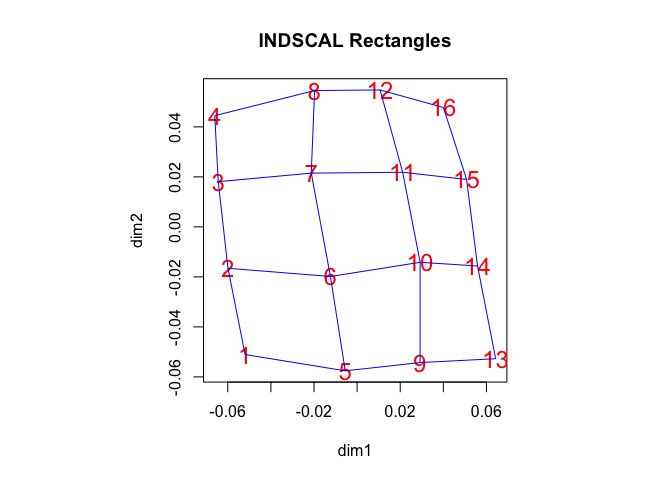Since there are only two different distance matrices we do not plot the $$T_k$$. For IDIOSCAL they are

## []
##               D1            D2
## D1  0.9969528775 -0.1401381035
## D2 -0.1900447368  1.0747955535
##
## []
##              D1           D2
## D1 1.0810614200 0.1374772239
## D2 0.1829126197 0.8261765584

and for INDSCAL we have

## []
##              [,1]        [,2]
## [1,] 0.8760383307 0.000000000
## [2,] 0.0000000000 1.312868518
##
## []
##             [,1]         [,2]
## [1,] 1.162505002 0.0000000000
## [2,] 0.000000000 0.8206214109

The $$T_k$$ from IDIOSCAL are heavily diagonally dominant, which explains why INDSCAL and IDIOSCAL are very similar.

For completeness we also give the NODIFF iterations.

h.nodiff <- smacofNODIFF (w, delta, res.diag$gspace) ## itel 1 sold Inf snew 191.485156 ## itel 2 sold 191.485156 snew 0.090857 ## itel 3 sold 0.090857 snew 0.089019 ## itel 4 sold 0.089019 snew 0.088337 ## itel 5 sold 0.088337 snew 0.087988 ## itel 6 sold 0.087988 snew 0.087767 ## itel 7 sold 0.087767 snew 0.087613 ## itel 8 sold 0.087613 snew 0.087499 ## itel 9 sold 0.087499 snew 0.087413 ## itel 10 sold 0.087413 snew 0.087348 ## itel 11 sold 0.087348 snew 0.087298 ## itel 12 sold 0.087298 snew 0.087260 ## itel 13 sold 0.087260 snew 0.087231 ## itel 14 sold 0.087231 snew 0.087209 ## itel 15 sold 0.087209 snew 0.087192 ## itel 16 sold 0.087192 snew 0.087179 ## itel 17 sold 0.087179 snew 0.087170 ## itel 18 sold 0.087170 snew 0.087162 ## itel 19 sold 0.087162 snew 0.087156 ## itel 20 sold 0.087156 snew 0.087152 ## itel 21 sold 0.087152 snew 0.087149 ## itel 22 sold 0.087149 snew 0.087146 ## itel 23 sold 0.087146 snew 0.087144 ## itel 24 sold 0.087144 snew 0.087143 ## itel 25 sold 0.087143 snew 0.087142 ## itel 26 sold 0.087142 snew 0.087141 It may be of somew interest that smacofNODIFF can also be used if there is only a single replication. In the rectangle example we simply use the average of the two matrices of dissimilarities. w <- list (w[]) delta <- list(perception[]+perception[]) s <- sum (w[] * delta[]^2) delta[] <- delta[] * sqrt (4.0 / s) h.single <- smacofNODIFF (w, delta, res.diag$gspace)
## itel     1 sold         Inf snew   87.722930
## itel     2 sold   87.722930 snew    0.047731
## itel     3 sold    0.047731 snew    0.045852
## itel     4 sold    0.045852 snew    0.045154
## itel     5 sold    0.045154 snew    0.044796
## itel     6 sold    0.044796 snew    0.044571
## itel     7 sold    0.044571 snew    0.044413
## itel     8 sold    0.044413 snew    0.044297
## itel     9 sold    0.044297 snew    0.044209
## itel    10 sold    0.044209 snew    0.044142
## itel    11 sold    0.044142 snew    0.044091
## itel    12 sold    0.044091 snew    0.044053
## itel    13 sold    0.044053 snew    0.044023
## itel    14 sold    0.044023 snew    0.044000
## itel    15 sold    0.044000 snew    0.043983
## itel    16 sold    0.043983 snew    0.043970
## itel    17 sold    0.043970 snew    0.043960
## itel    18 sold    0.043960 snew    0.043952
## itel    19 sold    0.043952 snew    0.043946
## itel    20 sold    0.043946 snew    0.043942
## itel    21 sold    0.043942 snew    0.043939
## itel    22 sold    0.043939 snew    0.043936
## itel    23 sold    0.043936 snew    0.043934
## itel    24 sold    0.043934 snew    0.043933
## itel    25 sold    0.043933 snew    0.043931
## itel    26 sold    0.043931 snew    0.043931

# 5 Example (Colors)

Our second, somewhat larger, example uses data from Helm (1959), also included in the smacof package. From the help file:

A detailed description of the experiment can be found in Borg and Groenen (2005), p. 451 with the corresponding Table 21.1. containing distance estimates for color pairs. There were 14 subjects that rated the similarity of colors, 2 of whom replicated the experiment. 10 subjects have a normal color vision (labelled by N1 to N10 in our list object), 4 of them are red-green deficient in varying degrees. In this dataset we give the dissimilarity matrices for each of the subjects, including the replications.

The NODIFF iterations are

h.diag <- smacofNODIFF (weights, delta, ind.helm$gspace) ## itel 1 sold Inf snew 624.988956 ## itel 2 sold 624.988956 snew 0.078972 ## itel 3 sold 0.078972 snew 0.078590 ## itel 4 sold 0.078590 snew 0.078483 ## itel 5 sold 0.078483 snew 0.078451 ## itel 6 sold 0.078451 snew 0.078441 ## itel 7 sold 0.078441 snew 0.078437 ## itel 8 sold 0.078437 snew 0.078436 ## itel 9 sold 0.078436 snew 0.078435 The IDIOSCAL iterations are: h.idio <- smacofIDIOSCAL (weights, delta, idi.helm$cweights, idi.helm$gspace) ## itel 1 sold Inf sa 632.304054 sb 0.063500 ## itel 2 sold 0.063500 sa 0.043978 sb 0.043829 ## itel 3 sold 0.043829 sa 0.043316 sb 0.043283 ## itel 4 sold 0.043283 sa 0.043092 sb 0.043083 ## itel 5 sold 0.043083 sa 0.043015 sb 0.043013 ## itel 6 sold 0.043013 sa 0.042989 sb 0.042988 ## itel 7 sold 0.042988 sa 0.042980 sb 0.042980 ## itel 8 sold 0.042980 sa 0.042977 sb 0.042977 ## itel 9 sold 0.042977 sa 0.042976 sb 0.042976 The INDSCAL iterations are: h.diag <- smacofINDSCAL (weights, delta, ind.helm$cweights, ind.helm$gspace) ## itel 1 sold Inf sa 631.986429 sb 0.065305 ## itel 2 sold 0.065305 sa 0.046937 sb 0.046808 ## itel 3 sold 0.046808 sa 0.046355 sb 0.046325 ## itel 4 sold 0.046325 sa 0.046131 sb 0.046123 ## itel 5 sold 0.046123 sa 0.046037 sb 0.046035 ## itel 6 sold 0.046035 sa 0.045995 sb 0.045993 ## itel 7 sold 0.045993 sa 0.045974 sb 0.045973 ## itel 8 sold 0.045973 sa 0.045963 sb 0.045962 ## itel 9 sold 0.045962 sa 0.045956 sb 0.045956 ## itel 10 sold 0.045956 sa 0.045953 sb 0.045952 ## itel 11 sold 0.045952 sa 0.045951 sb 0.045950 ## itel 12 sold 0.045950 sa 0.045949 sb 0.045949 ## itel 13 sold 0.045949 sa 0.045948 sb 0.045948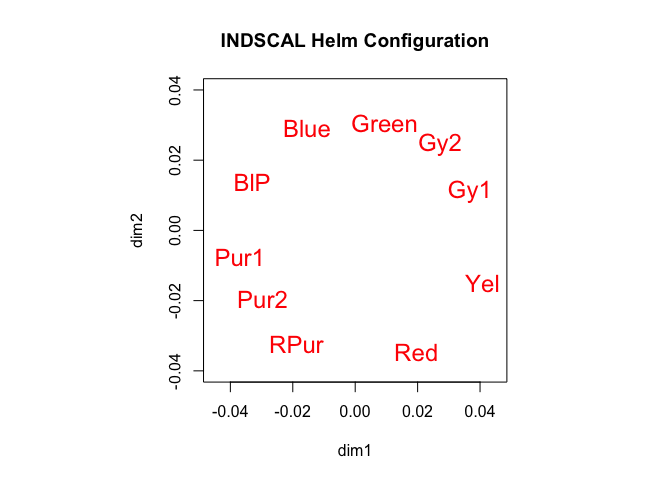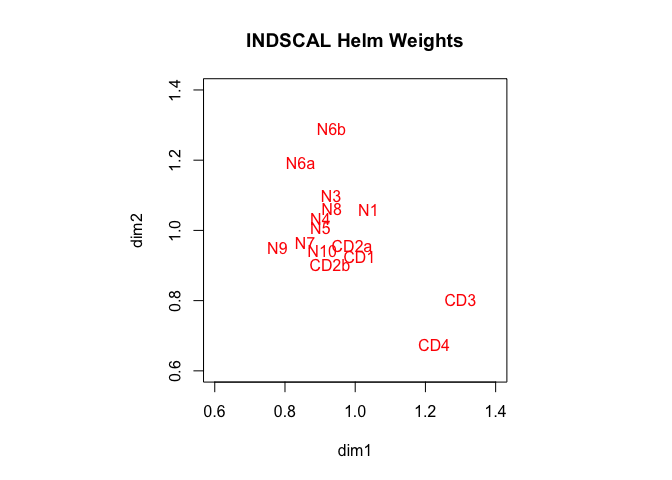# 6 Stability In this paper we study, in addition, the stability of IDMDS solutions. We define stability in the usual way. In inferential statistics we look at the second derivatives of the likelihood function at the maximum likelihood estimate. In MDS we look at the second derivatives of stress at a local minimum. Our results extend the results in De Leeuw (2017) from MDS to IDMDS. In general, if a function $$f:\mathbb{R}^n\Rightarrow\mathbb{R}$$ is two times continuously differentiable then $\begin{equation*} f(x)=f(y)+(x-y)'\mathcal{D}f(y)+\frac12(x-y)'\mathcal{D}^2f(y)(x-y)+o(\|x-y\|). \end{equation*}$ If $$f$$ has a local minimum in $$y$$ then $$\mathcal{D}f(y)=0$$ and $$\mathcal{D}^2f(y)\gtrsim 0$$. Consider all $$x$$ of the form $\begin{equation}\label{E:xform} x=\begin{bmatrix}x_1\\y_2\end{bmatrix}, \end{equation}$ where $$y_2$$ has the last $$n-m$$ elements of $$y$$. Then $\begin{equation} f(x)=f(y)+\frac12(x_1-y_1)'H(y)(x_1-y_1)+o(\|x_1-y_1\|), \end{equation}$ where $$H(y)$$ is the leading $$m\times m$$ submatrix of the $$\mathcal{D}^2f(y)$$. Thus the set of all $$x$$ of the form $$\eqref{E:xform}$$ with $$f(x)\leq(1+\epsilon)f(y)$$ can be approximated by choosing $$x_1$$ in the ellipsoid $\begin{equation} (x_1-y_1)'H(y)(x_1-y_1)\leq 2\epsilon f(y) \end{equation}$ We could choose $$\epsilon$$ to be $$0.1$$, for example, which means we look at a perturbation region where stress is at most $$10\%$$ larger than the minimum we have found. To apply these general results to IDMDS we need the second derivatives of stress. Of course these second derivatives can also be used for other purposes, such as checking the necessary conditions for a local minimum, or for implementations of Newton’s method. Start with the derivatives with repect to $$X$$. We have $\begin{equation} \frac{\partial^2 d_{ij}(X_k)}{\partial x\partial x}=\frac{1}{d_{ij}(X_k)}\left\{(C_k\otimes A_{ij})-\frac{(C_k\otimes A_{ij})xx'(C_k\otimes A_{ij})}{d_{ij}^2(X_k)}\right\}, \end{equation}$ and, of course, $\begin{equation} \frac{\partial^2 d_{ij}^2(X_k)}{\partial x\partial x}=C_k\otimes A_{ij}. \end{equation}$ Thus $\begin{equation} \frac{\partial^2\sigma(X_1,\cdots,X_m)}{\partial x\partial x}= V_\star^{\ }-H_\star^{\ }(x), \end{equation}$ where $\begin{equation} H_\star^{\ }(x)=\sum_{k=1}^m\mathop{\sum\sum}_{1\leq i<j\leq n}w_{ijk}\frac{\delta_{ijk}}{d_{ij}(X_k)}\left\{(C_k\otimes A_{ij})-\frac{(C_k\otimes A_{ij})xx'(C_k\otimes A_{ij})}{d_{ij}^2(X_k)}\right\}. \end{equation}$ We now look at the second derivatives with repect to the $$T_k$$. First the IDIOSCAL case, which has $\begin{equation} \frac{\partial^2 d_{ij}^2(X_k)}{\partial t_k\partial t}=I\otimes X'A_{ij}X, \end{equation}$ and $\begin{equation} \frac{\partial^2 d_{ij}(X_k)}{\partial t_k\partial t_k}=\frac{1}{d_{ij}(X_k)}\left\{(I\otimes X'A_{ij}X)-\frac{(I\otimes X'A_{ij}X)t_k^{\ }t_k'(I\otimes X'A_{ij}X)}{d_{ij}^2(X_k)}\right\}. \end{equation}$ $\begin{equation} \frac{\partial^2\sigma(X_1,\cdots,X_m)}{\partial t_k\partial t_k}=(I\otimes X'V_kX)-G_k(t_k), \end{equation}$ where $\begin{equation} G_k(t_k)=\mathop{\sum\sum}_{1\leq i<j\leq n}w_{ijk}\frac{\delta_{ijk}}{d_{ij}(X_k)}\left\{(I\otimes X'A_{ij}X)-\frac{(I\otimes X'A_{ij}X)t_k^{\ }t_k'(I\otimes X'A_{ij}X)}{d_{ij}^2(X_k)}\right\}. \end{equation}$ For the INDSCAL case we have the same formulas, but without the $$I\otimes$$ part. Thus $\begin{equation} \frac{\partial^2\sigma(X_1,\cdots,X_m)}{\partial t_k\partial t_k}=X'V_kX-G_k(t_k), \end{equation}$ where $\begin{equation} G_k(t_k)=\mathop{\sum\sum}_{1\leq i<j\leq n}w_{ijk}\frac{\delta_{ijk}}{d_{ij}(X_k)}\left\{ X'A_{ij}X-\frac{X'A_{ij}Xt_k^{\ }t_k'X'A_{ij}X}{d_{ij}^2(X_k)}\right\}. \end{equation}$ We should perhaps mention that the perturbation results for IDIOSCAL are limited by the fact that the representation of $$X_k$$ as $$XT_k$$ is far from unique. Besides the obvious, and rather harmless, unidentifiability due to translation and expansion, we also have $$XT_k=(XQ)(Q^{-1}T_k)$$ for any nonsingular $$Q$$. This suggests we should incorporate an identification condition such as $$T_1=I$$ in our equations. We haven’t done this yet. Until we have chosen the identification condition the stability analysis for IDIOSCAL is not yet available. Note that the identification condition is not needed for the SMACOF algorithm, but it is needed for the stability analysis. # 7 Example (Colours Stability) We now apply our second derivative information to the Helm INDSCAL analysis. First for the configuration $$X$$. par(pty="s") hh<-smacofDerivativesX (weights, delta, h.diag$b, h.diag$x) smacofEllipsesX (h.diag$x, hh$h, hh$s, .05, 1, 2)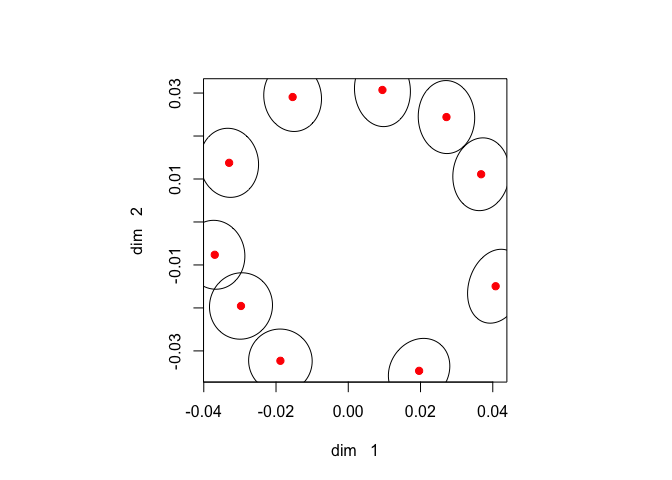For the weights $$T_k$$ in INDSCAL we compute a separate $$2\times 2$$ matrix of derivatives for each of the 16 replications.

bbb <- t(sapply (h.diag$b, diag)) hh <- array (0, c(2, 2, 16)) for (k in 1:16) { hk<-smacofDerivativesTKDiag (weights[[k]], delta[[k]], h.diag$b[[k]], h.diag$x) hh[, , k] <- hk$h
}
smacofEllipsesTDiag (bbb, hh, h.diag$s, .002, 1, 2)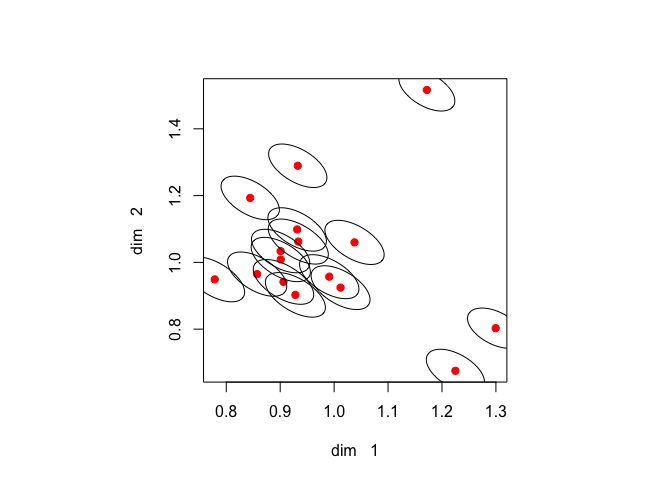It is remarkable that both the orientation and size of the ellipses are basically the same over the 16 replications. It remains to be seen if this is specific to this example or if it happens more generally. # 8 Projection In our stability analysis for $$X$$ we change row $$x_i$$, and we keep all other rows and all $$T_k$$ fixed and constant. As an alternative we could look at $\begin{equation} \sigma_\bullet(X)=\min_{T_1,\cdots,T_k}\sigma(X_1,\cdots,X_k). \end{equation}$ We project out the $$T_k$$ and loss becomes a function of $$X$$ only. Now $\begin{multline} \frac{\partial^2\sigma_\bullet(X)}{\partial x\partial x}=\frac{\partial^2\sigma(X_1,\cdots,X_k)}{\partial x\partial x}-\\\sum_{k=1}^m\frac{\partial^2\sigma(X_1,\cdots,X_k)}{\partial x\partial t_k} \left[\frac{\partial^2\sigma(X_1,\cdots,X_k)}{\partial t_k\partial t_k}\right]^+ \frac{\partial^2\sigma(X_1,\cdots,X_k)}{\partial t_k\partial x}. \end{multline}$ We have not implemented this alternative defintion of stability yet, mainly because it requires in addition the mixed second partials with respect to $$x$$ and the $$t_k$$. Clearly at a minimum $\begin{equation} \frac{\partial^2\sigma_\bullet(X)}{\partial x\partial x}\lesssim\frac{\partial^2\sigma(X_1,\cdots,X_k)}{\partial x\partial x}, \end{equation}$ which implies the perturbation ellipsoids will be larger. # 9 Nonmetric MDS In our results so far we have treated the $$\delta_{ijk}$$ as fixed constants. In Nonmetric Multidimensional Scaling (NMDS), however, stress can be defined as $\begin{equation}\label{E:stressnm} \sigma(X_1,\cdots,X_m)=\frac12\sum_{k=1}^m\min_{\delta_k\in\Delta_k}\mathop{\sum\sum}_{1\leq i<j\leq n}w_{ijk}(\delta_{ijk}-d_{ij}(X_k))^2, \end{equation}$ which makes the dissimilarities a function of the $$X_k$$. This projection changes the perturbation results for the $$X_k$$, in the same way as the result in the previous section did. But with NMDS there is an additional complication. Typically the dissimilarities are minimized over the polyhedral cones that define monotone regression. Although the partial minimum of stress over the cone is differentiable with respect to the $$X_k$$, it is not twice differentiable, because the monotone regression transformation itself is not differentiable. Again, we have chosen not to implement the resulting complications (yet). The perturbation results we have can be applied in NMDS, but the perturbation regions must be interpreted in terms of moving the points in the configuration for a given set of dissimilarties, which can of course be the optimal dissimilarities computed by NMDS. # 10 Appendix: Code ## 10.1 smacofIDIOSCAL.R # w is list of length m with n x n matrices # delta is list of length m with n x n matrices # b is list of length m with p x p matrices # x is n x p smacofIDIOSCAL <- function (w, delta, b, x, itmax = 100, eps = 1e-6, verbose = TRUE) { n <- nrow (x) p <- ncol (x) m <- length (w) itel <- 1 sold <- Inf repeat { sa <- 0.0 v <- matrix (0, n * p, n * p) u <- matrix (0, n * p, n * p) for (k in 1:m) { cmat <- tcrossprod (b[[k]]) xmat <- x %*% b[[k]] dmat <- as.matrix (dist (xmat)) vmat <- -w[[k]] diag(vmat) <- -rowSums(vmat) bmat <- -delta[[k]] * ifelse (dmat == 0, 0, 1 / dmat) diag(bmat) <- -rowSums(bmat) sa <- sa + sum (w[[k]] * (delta[[k]] - dmat) ^ 2) / 2.0 v <- v + kronecker (cmat, vmat) u <- u + kronecker (cmat, bmat) } x <- matrix (ginv (v) %*% u %*% as.vector (x), n, p) sb <- 0.0 for (k in 1:m) { xmat <- x %*% b[[k]] dmat <- as.matrix (dist (xmat)) vmat <- -w[[k]] diag(vmat) <- -rowSums(vmat) hmat <- crossprod (x, vmat %*% x) bmat <- -delta[[k]] * ifelse (dmat == 0, 0, 1 / dmat) diag(bmat) <- -rowSums(bmat) gmat <- crossprod (x, bmat %*% x) sb <- sb + sum (w[[k]] * (delta[[k]] - dmat) ^ 2) / 2.0 kmat <- ginv (hmat) %*% gmat for (j in 1:p) { b[[k]][, j] <- kmat %*% b[[k]][, j] } } if (verbose) { cat( "itel ", formatC (itel, digits = 3, format = "d"), "sold ", formatC ( sold, digits = 6, width = 10, format = "f" ), "sa ", formatC ( sa, digits = 6, width = 10, format = "f" ), "sb ", formatC ( sb, digits = 6, width = 10, format = "f" ), "\n" ) } if ((itel == itmax) || (sold - sb < eps)) break sold <- sb itel <- itel + 1 } return (list (x = x, b = b, s = sb)) } ## 10.2 smacofINDSCAL.R # w is list of length m with n x n matrices # delta is list of length m with n x n matrices # b is list of length m with p x p matrices # x is n x p smacofINDSCAL <- function (w, delta, b, x, itmax = 100, eps = 1e-6, verbose = TRUE) { n <- nrow (x) p <- ncol (x) m <- length (w) itel <- 1 sold <- Inf repeat { sa <- 0.0 v <- matrix (0, n * p, n * p) u <- matrix (0, n * p, n * p) for (k in 1:m) { cmat <- tcrossprod (b[[k]]) xmat <- x %*% b[[k]] dmat <- as.matrix (dist (xmat)) vmat <- -w[[k]] diag(vmat) <- -rowSums(vmat) bmat <- -delta[[k]] * ifelse (dmat == 0, 0, 1 / dmat) diag(bmat) <- -rowSums(bmat) sa <- sa + sum (w[[k]] * (delta[[k]] - dmat) ^ 2) / 2.0 v <- v + kronecker (cmat, vmat) u <- u + kronecker (cmat, bmat) } x <- matrix (ginv (v) %*% u %*% as.vector (x), n, p) sb <- 0.0 for (k in 1:m) { xmat <- x %*% b[[k]] dmat <- as.matrix (dist (xmat)) vmat <- -w[[k]] diag(vmat) <- -rowSums(vmat) hmat <- crossprod (x, vmat %*% x) bmat <- -delta[[k]] * ifelse (dmat == 0, 0, 1 / dmat) diag(bmat) <- -rowSums(bmat) gmat <- crossprod (x, bmat %*% x) sb <- sb + sum (w[[k]] * (delta[[k]] - dmat) ^ 2) / 2.0 kmat <- ginv (hmat) %*% gmat b[[k]] <- diag (drop (kmat %*% diag (b[[k]]))) } if (verbose) { cat( "itel ", formatC (itel, digits = 3, format = "d"), "sold ", formatC ( sold, digits = 6, width = 10, format = "f" ), "sa ", formatC ( sa, digits = 6, width = 10, format = "f" ), "sb ", formatC ( sb, digits = 6, width = 10, format = "f" ), "\n" ) } if ((itel == itmax) || (sold - sb < eps)) break sold <- sb itel <- itel + 1 } return (list (x = x, b = b, s = sb)) } ## 10.3 smacofDerivatives.R smacofDerivativesX <- function (w, delta, b, x) { n <- nrow (x) p <- ncol (x) m <- length (w) s <- 0.0 v <- matrix (0, n * p, n * p) u <- matrix (0, n * p, n * p) r <- matrix (0, n * p, n * p) for (k in 1:m) { cmat <- tcrossprod (b[[k]]) xmat <- x %*% b[[k]] dmat <- as.matrix (dist (xmat)) for (i in 1:(n - 1)) { for (j in (i + 1):n) { ei <- ifelse (i == 1:n, 1, 0) ej <- ifelse (j == 1:n, 1, 0) amat <- outer (ei - ej, ei - ej) kmat <- kronecker (cmat, amat) kx <- drop (kmat %*% as.vector(x)) kxkx <- outer (kx, kx) s <- s + w[[k]][i, j] * (delta[[k]][i, j] - dmat [i, j]) ^ 2 v <- v + w[[k]][i, j] * kmat u <- u + w[[k]][i, j] * (delta[[k]][i, j] / dmat[i, j]) * kmat r <- r + w[[k]][i, j] * (delta[[k]][i, j] / (dmat [i, j] ^ 3)) * kxkx } } } g <- drop ((v - u) %*% as.vector(x)) h <- v - u + r return (list (s = s, g = 0, h = h)) } smacofDerivativesTKDiag <- function (w, delta, b, x) { n <- nrow (x) p <- ncol (x) s <- 0.0 v <- matrix (0, p, p) u <- matrix (0, p, p) r <- matrix (0, p, p) cmat <- tcrossprod (b) xmat <- x %*% b dmat <- as.matrix (dist (xmat)) for (i in 1:(n - 1)) { for (j in (i + 1):n) { ei <- ifelse (i == 1:n, 1, 0) ej <- ifelse (j == 1:n, 1, 0) amat <- outer (ei - ej, ei - ej) kmat <- crossprod(x, amat %*% x) kx <- drop (kmat %*% diag(b)) kxkx <- outer (kx, kx) s <- s + w[i, j] * (delta[i, j] - dmat [i, j]) ^ 2 v <- v + w[i, j] * kmat u <- u + w[i, j] * (delta[i, j] / dmat[i, j]) * kmat r <- r + w[i, j] * (delta[i, j] / (dmat[i, j] ^ 3)) * kxkx } } g <- drop ((v - u) %*% diag(b)) h <- v - u + r return (list (s = s, g = g, h = h)) } ## 10.4 smacofEllipses.R smacofEllipsesX <- function (x, h, f, eps, s = 1, t = 2) { n <- nrow (x) plot ( x, col = "RED", pch = 21, bg = "RED", xlab = paste("dim ", formatC( s, digits = 1, format = "d" )), ylab = paste("dim ", formatC( t, digits = 1, format = "d" )) ) z <- cbind (sin (seq(-pi, pi, length = 100)), cos (seq(-pi, pi, length = 100))) * sqrt(2 * eps * f) for (i in 1:n) { ii <- c((s - 1) * n + i, (t - 1) * n + i) y <- x[i, c(s, t)] amat <- h[ii, ii] heig <- eigen (amat) zi <- z %*% diag (1 / sqrt (heig$values))
zi <- tcrossprod(zi, heig$vectors) zi <- zi + matrix (y, 100, 2, byrow = TRUE) lines (list(x = zi[, 1], y = zi[, 2])) } } smacofEllipsesTDiag <- function (b, h, f, eps, s = 1, t = 2) { par (pty= "s") m <- nrow (b) ii <- c(s, t) plot ( b[, ii], col = "RED", pch = 21, bg = "RED", xlab = paste("dim ", formatC( s, digits = 1, format = "d" )), ylab = paste("dim ", formatC( t, digits = 1, format = "d" )) ) z <- cbind (sin (seq(-pi, pi, length = 100)), cos (seq(-pi, pi, length = 100))) * sqrt(2 * eps * f) for (k in 1:m) { y <- b[k, ii] amat <- h[ii, ii, k] heig <- eigen (amat) zi <- z %*% diag (1 / sqrt (heig$values))
zi <- tcrossprod(zi, heig\$vectors)
zi <- zi + matrix (y, 100, 2, byrow = TRUE)
lines (list(x = zi[, 1], y = zi[, 2]))
}
}